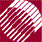ECE532 Biomedical Optics © 1998 Steven L. Jacques, Scott A. Prahl Oregon Graduate Institute

Photon hops to new position

The photon takes a step of variable length determined by sampling the exponential probability density function for the stepsize (s) of movement before the photon interacts with the tissue by the combination of absorption and scattering:

p(s) = µtexp(-µts)

whose average value is s = 1/µt where µt = µa + µs. A random number (rnd) is specified and used to sample p(s):

s = -log(rnd)/(mua + mus);

The current position (x, y, z) is updated by the projections of s onto each of the x, y, and z axes. For example, x += s * us means x = x + s*us.

/**** HOP
Take step to new position
s = stepsize
ux, uy, uz specify current photon trajectory
*****/
while ((rnd = RandomNum) < = 0.0);   /* yields 0 < rnd < = 1 */
s = -log(rnd)/(mua + mus);          /* Step size.  Note: log() is base e */
x += s * ux;                        /* Update positions. */
y += s * uy;
z += s * uz;

to next page | Chapter 4 | Course | Home

©1998, Steven L. Jacques, Scott A. Prahl, Oregon Graduate Institute, Oregon Medical Laser Center